# Count Unique Values in Excel

## Counting unique values in Excel

Summary:

One of the most common requirements of data manipulation is to either remove duplicates from the data or get the unique count of values. Although, excel doesn’t provide any default function to get the unique count of values but still the removing of duplicate values and getting the unique count of values can be done easily in Excel.

There are majorly two ways to get the unique count of values:

• To get the unique count of values using the advanced filter option
• Count the number of unique values by using excel functions

### To get the unique count of values using the advanced filter option

In this method user can use the “Advanced Filter” option to get the unique values from the data, paste them into a new location and then use the excel “count” function to get the required result

Following are the steps to get the unique count:

1. Select the range of the data in the column for which you want to get the unique count2. On the Data tab, under Sort & Filter group, click Advanced. The Advanced Filter dialog box appears.3. Select Copy to another location option4. In the Copy to: box enter the cell reference. For now, I have given a cell reference as C25. Select the Unique records only check box, and click  The result of this would be that we will get the unique list of all the names in new column i.e. Column C (as shown)6. Now, use the excel function “COUNT/COUNTA” on column C values to get the unique count of the values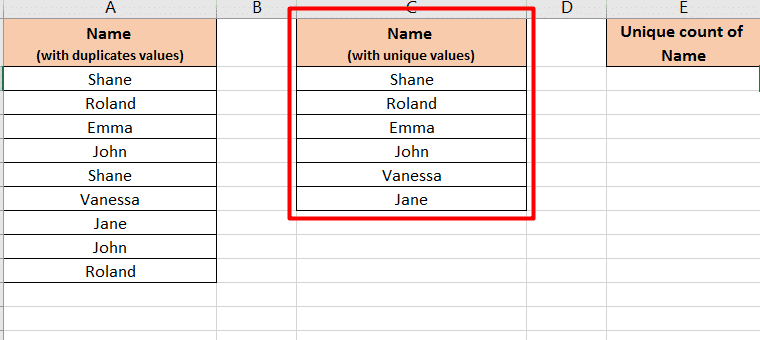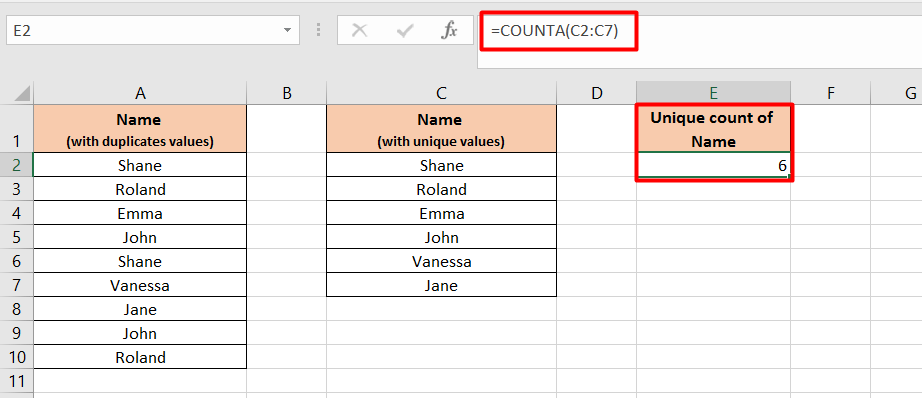### Count the number of unique values by using Excel functions

As we discussed above, there is no direct built-in function in excel which gives distinct count values. But, using different functions together like “IF”, “SUM”, “FREQUENCY”, “LEN”, “MATCH” etc., we can get the unique count of values.

Following are different scenarios in which we can use the different functions together to the unique count of the values:

1. Count the unique “number” values in cells, but does not count “blank” cells or “text” values: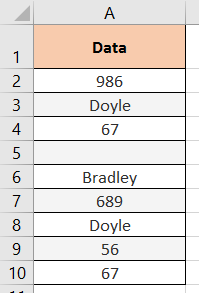Consider that we have a list of values which contains “numbers”, “text” and “blanks”

Now, to get the unique count of only numbers within that list we can use a formula:

` SUM(IF(FREQUENCY(A2:A10,A2:A10)>0,1))`1. Count the unique “number” and “text” values in cells which do not contain blank: Consider that we have a list of values which contains “numbers” and “text”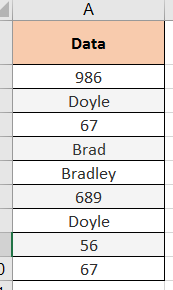Now, to get the unique count of both numbers and text values within that list we can use a formula:

` {SUM(IF(FREQUENCY(MATCH(A2:A10,A2:A10,0),MATCH(A2:A10,A2:A10,0))>0,1))}`1. Count the unique “number” and “text” values in cells but does not count blank cells: Consider that we have a list of values which contains “numbers”, “text” and “blank” cells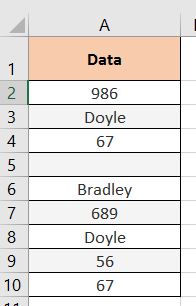Now, to get the unique count of both numbers and text values within that list we can use a formula:

`{SUM(IF(FREQUENCY(IF(LEN(A2:A10)>0,MATCH(A2:A10,A2:A10,0),""), IF(LEN(A2:A10)>0,MATCH(A2:A10,A2:A10,0),""))>0,1))}`